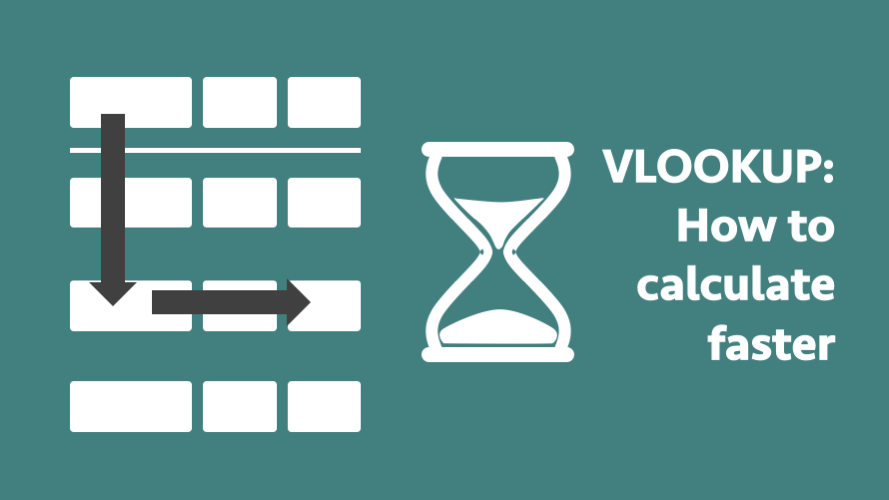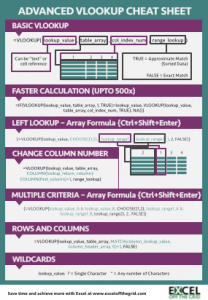Get our FREE VBA eBook of the 30 most useful Excel VBA macros.

Automate Excel so that you can save time and stop doing the jobs a trained monkey could do.

# VLOOKUP: How to calculate fasterI hardly ever use an approximate match with VLOOKUP due to the risk of getting an incorrect result.  But I learned this little trick – which provided almost unbelievable speed increases.

## The problem

With a VLOOKUP an exact match (i.e. including FALSE as the last argument) is a slow method of calculation.  It will search through each record one-by-one until it finds a match.  Using an approximate match (i.e. including TRUE as the last argument) is very fast in comparison, as it does a binary search.  An approximate match only works with sorted data but can find the lookup value faster.  However, if the lookup value is not in the list it will return an incorrect result.

## The solution

This trick involves combining two approximate matches together to return the equivalent of an exact match.  If there is no matched value it will return an #N/A result.

An exact match VLOOKUP would be defined like this:

`=VLOOKUP(lookup_value, table_array, col_index_num, FALSE)`

But combining two VLOOKUP’s to create an equivalent to an exact match looks like this:

```=IF(VLOOKUP(lookup_value, table_array, 1, TRUE)=lookup_value,
VLOOKUP(lookup_value, table_array, col_index_num, TRUE),NA())```

## How does this work?

Let’s break this formula down into understandable sections.  First, Let’s consider the first VLOOKUP statement.

```=IF(VLOOKUP(lookup_value, table_array, 1, TRUE)=lookup_value,
VLOOKUP(lookup_value, table_array, col_index_num, TRUE),NA())```

The section highlighted above carries out an approximate match VLOOKUP calculation on the first column.   If it finds an exact match it will return the lookup value, otherwise it will return a different value.

Now, let’s consider the IF statement.

```=IF(VLOOKUP(lookup_value, table_array, 1, TRUE)=lookup_value,
VLOOKUP(lookup_value, table_array, col_index_num, TRUE),NA())```

The returned value is compared to the original lookup value.  If it is the same (i.e. an exact match exists) it will become TRUE, if it is different it will become FALSE.

Finally, let’s look at the second VLOOKUP.

```=IF(VLOOKUP(lookup_value, table_array, 1, TRUE)=lookup_value,
VLOOKUP(lookup_value, table_array, col_index_num, TRUE),NA())```

Where the value of the first VLOOKUP is TRUE the second VLOOKUP is then calculated.  We already know that an exact match exists, so it will return the correct value from the column number identified.

## The benefit

This formula may seem like an over-complication.  If your worksheet is calculating quickly, then Yes, it probably is.  However, a slow calculating worksheet can waste a lot of time.  So, if your worksheet is slow, this formula can create significant speed benefits.  Even though the new formula is carrying out two VLOOKUPs and an IF, the calculation speed is still much faster than a single exact match VLOOKUP.

I carried out a few speed tests.  Using 100,000 lines of unique data, I timed a VLOOKUP to find 5,000 lines matching lines.  Average times over 5 tests were:

 Seconds Exact Match 14.6107 Combined Approximate Match 0.0267

Wow!  That’s over 500 times faster! 500 times faster!!  That is equivalent to an 8-minute calculation being reduced down to just 1 second.  Don’t tell me you’re not blown away by this, because I was.

Jeff Weir at Daily Dose of Excel has carried out even more tests to find fast this trick is.  Check out his post, as it is very interesting, especially the chart comparing speed as the volume of data increases.

## Conclusion

Are you are using a lot of VLOOKUPs?  Is the calculation speed feeling a bit sluggish?  If so, sort your data and switch to this VLOOKUP combination with approximate matches.  You will see an instant speed boost.  I can’t guarantee it will be the same speed boost I obtained, but you should definitely see a speed increase.

Download the Advanced VLOOKUP Cheat Sheet.  It includes most of the tips and tricks we’ve covered in this series, including faster calculations, multiple criteria, left lookup and much more.## Other posts in the Mastering VLOOKUP Series

Hey, I’m Mark, and I run Excel Off The Grid.

My parents tell me that at the age of 7 I declared I was going to become a qualified accountant. I was either psychic or had no imagination, as that is exactly what happened. However, it wasn't until I was 35 that my journey really began.

In 2015, I started a new job, for which I was regularly working after 10pm. As a result, I rarely saw my children during the week. So, I started searching for the secrets to automating Excel. I discovered that by building a small number of simple tools, I could combine them together in different ways to automate nearly all my regular tasks. This meant I could work less hours (and I got pay raises!). Today, I teach these techniques to other professionals in our training program so they too can spend less time at work (and more time with their children and doing the things they love).

I'm guessing the examples in this post don't exactly match your situation. We all use Excel differently, so it's impossible to write a post that will meet everybody's needs. By taking the time to understand the techniques and principles in this post (and elsewhere on this site), you should be able to adapt it to your needs.

But, if you're still struggling you should:

1. Read other blogs, or watch YouTube videos on the same topic. You will benefit much more by discovering your own solutions.
2. Ask the 'Excel Ninja' in your office. It's amazing what things other people know.
3. Ask a question in a forum like Mr Excel, or the Microsoft Answers Community. Remember, the people on these forums are generally giving their time for free. So take care to craft your question, make sure it's clear and concise.  List all the things you've tried, and provide screenshots, code segments and example workbooks.
4. Use Excel Rescue, who are my consultancy partner. They help by providing solutions to smaller Excel problems.

What next?
Don't go yet, there is plenty more to learn on Excel Off The Grid.  Check out the latest posts:

## 2 thoughts on “VLOOKUP: How to calculate faster”

1.Moshe Braner says:

This approach can also get rid of the annoying #N/A wherever a match is not found – which I often later search-and-replace with blanks. Just change the last parameter in the formula from NA() to whatever you want, e.g., “”. There must be additional ways to get rid of that #N/A?

•Excel Off The Grid says:

IFERROR is the best function to remove the #N/A error.